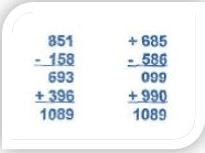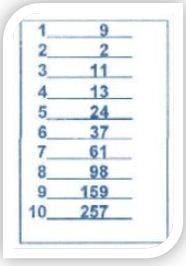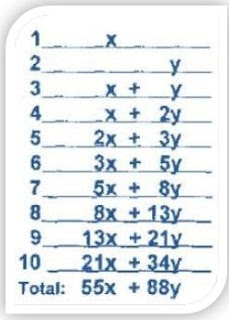In this mathemagics workbook, I am going to show you simple logic roots of maths tricks. This is the only one of the way of maths tricks and tutorial you will Learn math magic tricks and methods easily. Once you know about math facts You can make your brain faster like a computer and also math magic tricks Amaze Your Friends and Play With maths tricks With Numbers and maths tricks for fast calculation,mathemagicsworkbook.com## Thursday, June 13, 2019SAT MATH TRICKS

## INTRODUCTORY ALGEBRA AND SAT MATH TRICKS

ALGEBRA AND SAT MATH TRICKS | want to learn SAT MATH TIPS ! So what! Let's Play with numbers. I find that arithmetic can Be as entertaining as magic. But to understand the MATHS TRICKS magical secrets of arithmetic.requires algebra, Of course, there are other reasons to learn algebra (SAT, modeling real-world problems, computer programming, to name just a few), but what called me first interested in algebra was the desire to understand some mathematical magic tricks that Now I present to you!

## SAT MATH TOPICS

CHAPTER 6

### PSYCHIC MATHEMATICS

Tell a volunteer from the audience, "Think of a number, any number, but to do
It’s easy for you, "I should say," think of a 1 or 2 digit number. "After
He reminded his volunteer that there is no way to know his number, ask him to:

1. Double the number.
3. Divide the total by 2.
4. Subtract the original number.

Then: "Was the answer you got by chance number SIX?" Try this
In yourself first and you'll see that the sequence always produces the number 6 without
It matters what number was originally selected.

For example, if the original number is 15.
1. 2x15 = 30
2. 30 + 12 = 42
3. 4 2/2 = 21
4. 21-15 = 6

#### Why this SAT trick works

Let's represent the original number by the letter y. Here are the functions that
Done in the order in which you made them:

12 and
2. 2y + 12
3. (2y + 12) / 2 = y + 6
4. y + 6 - y = 6

So don’t worrier what number your a volunteer chooses, the final answer will always be

6. If you repeat this trick, have the volunteer add a different number in step 2 (say 18).
The final answer will be half that number (2 x 15 = 30; 30 + 18 = 48; 48 + 2 = 24; 24 - 15= 9). MAGIC 1089!

Have the member of your audience take out a piece of paper and pencil and:

1. Secretly write a 3-digit number in which the first digit is larger than the
last digit.

2. Invert that number and subtract it from the first number. If the result is
Number of 2 digits; tell them to put a 0 in front.

At the end of this sequence, your answer, 1089, will appear magically, it does not matter What number did you originally choose? For example:### Why this trick works

No matter the 3-digit number that you or anyone else chooses in this game, the final
The result will always be in 1089. Why? Let abc denote the unknown 3-digit number.
Algebraically, this equals:When you invert the number and subtract it from the original number, you get them
ABC number, algebraically equal to:Therefore, after subtracting in step 2, we must have one of the following multiples of 99: 099, 198, 297, 396, 495, 594, 693, 792 or 891, each of which will produce 1089 after adding it upside down from itself, as we did in step 3.

This trick combines a quick mental calculation with a surprising prediction. Giving the viewer a card with ten lines, numbered from 1 to 10, make the viewer think of 2 positive numbers between 1 and 20, and enter them on lines 1 and 2 of the card. Then have the viewer write the sum of lines 1 and 2 on line 3, then the sum of lines 2 and 3 online 4, and so on, as illustrated below.WHY THIS SAT WORKS

To perform the quick calculation, simply multiply the number on line 7 by 11. Here 61 x 11 = 671. The reason why this works is illustrated in the following table. If we denote the numbers on lines 1 and 2 with x and y respectively, then the sum of lines 1 up to 10 must be 55x + 88y, which is equivalent to 11 times (5x + 8y), that is, eleven times the number on line 7. As for the prediction, we exploit the fact that for any positive number,### MATHEMATICS AND MATHEMATICS OF SAT.

Mastering math can help students take the SAT, a required entrance exam many universities Even in its latest version, the mathematical reasoning section of the SAT.
It still requires an understanding of basic arithmetic but does not ask questions that require Long tedious calculations. In fact, he is even allowed (and recommended) to use a calculator during the exam. There is a greater emphasis on mathematical reasoning instead of laborious computing. The Mathematics user is encouraged to explore creativity.

Solutions to problems. Look at your numbers. Are there special characteristics of the problem?

#### What can you exploit?

As an introduction, can you see a smart way to add?

Do not let the parentheses fool you. Since the sum is associative, we can rewrite the Problem like

Notice how the numbers will cancel each other out, and all we have left is

Here is one for you to try. Simplify:

Notice how the 3, 5, 7, 9 and 11 cancel each other, which leaves us with 1/3 Although the SAT also covers topics such as algebra and geometry, it has a numerical sense is invaluable. Do your answers seem reasonable? With  Mathemagics, I hope we have given you a greater numerical sense.

RELATED

TAGS-sat math topics, sat maths pdf, sat math formulas, sat math practice worksheets, sat math tips, khan academy sat math, new sat math topics